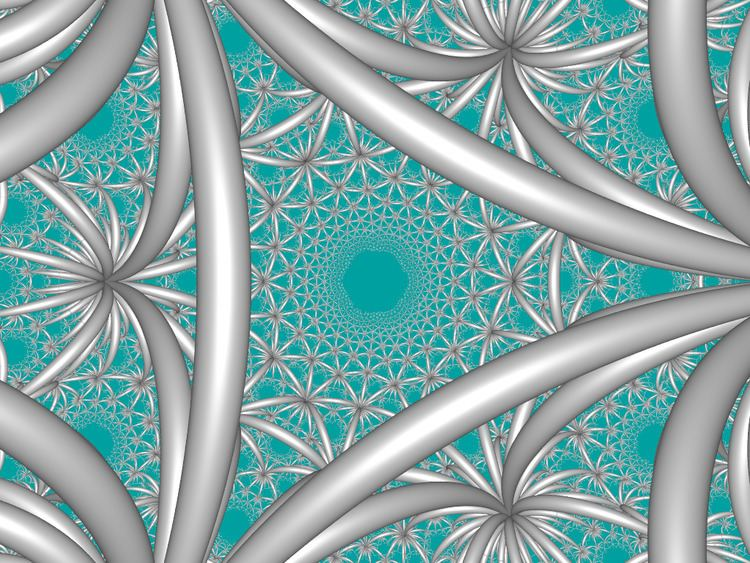# Triangular tiling honeycomb

Updated on
Covid-19The triangular tiling honeycomb is one of 11 paracompact regular space-filling tessellations (or honeycombs) in hyperbolic 3-space. It is called paracompact because it has infinite cells and vertex figures, with all vertices as ideal points at infinity. It has Schläfli symbol {3,6,3}, being composed of triangular tiling cells. Each edge of the honeycomb is surrounded by three cells, and each vertex is ideal with infinitely many cells meeting there. Its vertex figure is a hexagonal tiling.

## Contents

A geometric honeycomb is a space-filling of polyhedral or higher-dimensional cells, so that there are no gaps. It is an example of the more general mathematical tiling or tessellation in any number of dimensions.

Honeycombs are usually constructed in ordinary Euclidean ("flat") space, like the convex uniform honeycombs. They may also be constructed in non-Euclidean spaces, such as hyperbolic uniform honeycombs. Any finite uniform polytope can be projected to its circumsphere to form a uniform honeycomb in spherical space.

## Symmetry

It has two lower reflective symmetry constructions, as an alternated order-6 hexagonal tiling honeycomb, , and as from , which alternates 3 types (colors) of triangular tilings around every edge. In Coxeter notation, the removal of the 3rd and 4th mirrors, [3,6,3*] creates a new Coxeter group [3[3,3]], , subgroup index 6. The fundamental domain is 6 times larger. By Coxeter diagram there are 3 copies of the first original mirror in the new fundamental domain: .

## Images

It is similar to the 2D hyperbolic infinite-order apeirogonal tiling, {∞,∞} with infinite apeirogonal faces and all vertices are on the ideal surface.

## Related honeycombs

It is one of 15 regular hyperbolic honeycombs in 3-space, 11 of which like this one are paracompact, with infinite cells or vertex figures.

There are nine uniform honeycombs in the [3,6,3] Coxeter group family, including this regular form as well as the bitruncated form, t1,2{3,6,3}, with all truncated hexagonal tiling facets.

## Rectified triangular tiling honeycomb

The rectified triangular tiling honeycomb, , has alternating trihexagonal tiling and hexagonal tiling cells, with a triangular prism vertex figure.

## Symmetry

A lower symmetry of this honeycomb can be constructed as a cantic order-6 hexagonal tiling honeycomb, . A second lower construction, index is .

## Truncated triangular tiling honeycomb

The truncated triangular tiling honeycomb,, is the same as the hexagonal tiling honeycomb, .

## Bitruncated triangular tiling honeycomb

The bitruncated triangular tiling honeycomb, , has truncated hexagonal tiling cells, with a tetrahedral vertex figure.

## Cantellated triangular tiling honeycomb

The cantellated triangular tiling honeycomb, , has truncated hexagonal tiling and triangular prism cells, with a triangular prism vertex figure.

## Symmetry

It can also be constructed as a half-symmetry form, cantic snub triangular tiling honeycomb, , symmetry [3+,6,3].

## Cantitruncated triangular tiling honeycomb

The cantitruncated triangular tiling honeycomb, , has rhombitrihexagonal tiling and hexagonal prism cells, with a tetrahedron vertex figure.

## Runcinated triangular tiling honeycomb

The runcinated triangular tiling honeycomb, , has hexagonal tiling and triangular prism cells, with a hexagonal antiprism vertex figure.

## Runcitruncated triangular tiling honeycomb

The runcitruncated triangular tiling honeycomb, , has hexagonal tiling, rhombitrihexagonal tiling, triangular prism, and dodecagonal prism cells.

## Omnitruncated triangular tiling honeycomb

The omnitruncated triangular tiling honeycomb, , has truncated trihexagonal tiling and hexagonal prism cells, with a tetrahedron vertex figure.

## Runcisnub triangular tiling honeycomb

The runcisnub triangular tiling honeycomb, , has trihexagonal tiling, triangular tiling, triangular prism, and triangular cupola cells. It is vertex-transitive, but not uniform, since it contains Johnson solid triangular cupola cells.

## References

Similar Topics
The War (2007 TV series)
Jamie Borger
Babá Ken Okulolo
Topics